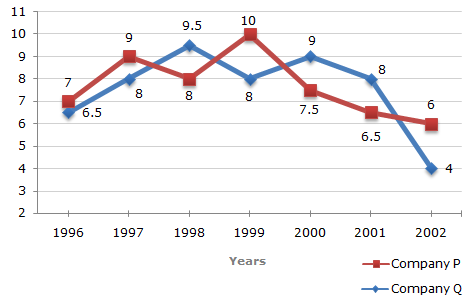# Data Interpretation - Line Charts - Discussion

### Discussion :: Line Charts - Line Chart 8 (Q.No.2)

Two different finance companies declare fixed annual rate of interest on the amounts invested with them by investors. The rate of interest offered by these companies may differ from year to year depending on the variation in the economy of the country and the banks rate of interest. The annual rate of interest offered by the two Companies P and Q over the years are shown by the line graph provided below.

Annual Rate of Interest Offered by Two Finance Companies Over the Years.2.

If two different amounts in the ratio 8:9 are invested in Companies P and Q respectively in 2002, then the amounts received after one year as interests from Companies P and Q are respectively in the ratio?

 [A]. 2:3 [B]. 3:4 [C]. 6:7 [D]. 4:3

Explanation:

Let the amounts invested in 2002 in Companies P and Q be Rs. 8x and Rs. 9x respectively.

Then, interest received after one year from Company P = Rs. (6% of 8x)
 = Rs. 48 x. 100
and interest received after one year from Company Q = Rs. (4% of 9x)
 = Rs. 36 x. 100Required ratio =48 x100
= 4 .36 x100
3

 Ashutosh said: (Jul 9, 2011) I think the answer given here is wrong. Because the ratio is asked for the amount after one year, whereas in solution they are comparing the ratio of interest. Some one please help me if I'm wrong.

 Reshmi said: (Dec 10, 2012) Ratio asked for amount received after one year AS INTEREST.

 Anna said: (May 27, 2014) But we receive 1.06 after one year I do not understand why the ratio is times 6% instead of 1+6%.

 Om Prakash Mahto said: (May 31, 2014) This answer is correct. And in fact this can be solved in a shorter way, this problem is similar to that of PARTNERSHIP. (8 x 6) / (9 x 4). Because the amount will be equally distributed as per the share and that of their respective interest.

 Navin Mallesh said: (Feb 15, 2018) @Ashutosh. The question is for a year however ratio remains same even the amount is changing. Ex = 4/3*12/12 = 4/3.

 Vikas said: (Sep 1, 2018) company P : company Q. Amount = 8 : 9 Interest rate = 6 : 4 So calculate - 8 : 9 = 6 : 4. 8/9 = 6/4. Result = 4/3.

 Manas M said: (Aug 26, 2021) 6 * 8/4 * 3 = 4/3.

 Mohan Prakash said: (Jan 10, 2022) The answer is correct only if the invested amount is same. If they are different they can't cancel each other and returns some value. Thus the ratio 4/3* (7/9) like some value would be returned.